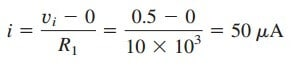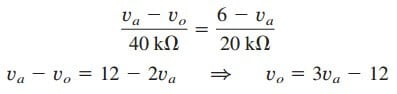# Inverting Op Amp Equation – Inverting Operational AmplifierWe will encounter the two types of amplifiers. One of them is inverting operational amplifier. Thus, it is important to learn it especially the inverting op amp equation.

## Inverting Op Amp Equation

In this post, we consider some useful op amp circuits that often serve as modules for designing more complex circuits. The first of such op amp circuits is the inverting amplifier shown in Figure.(1).

In this circuit, the non-inverting input is grounded, vi is connected to the inverting input through R1, and the feedback resistor Rf is connected between the inverting input and output. Our goal is to obtain the relationship between the input voltage vi and the output voltage vo.

Applying KCL at node 1,

But v1 = v2 = 0 for an ideal op amp, since the non-inverting terminal is
grounded. Hence,

The voltage gain is Av = vo/vi = –Rf/R1. The designation of the circuit in
Figure.(1) as an
inverter arises from the negative sign. Thus,

An inverting amplifier reverses the polarity of the input signal while amplifying it.

Notice that the gain is the feedback resistance divided by the input resistance which means that the gain depends only on the external elements connected to the op amp. In view of Equation.(2), an equivalent circuit for the inverting amplifier is shown in Figure.(2). The inverting amplifier is used, for example, in a current-to-voltage converter.

## Inverting Op Amp Equation Example

1. Refer to the op amp in Figure.(3). If vi = 0.5 V, calculate: (a) the output voltage vo, and (b) the current in the 10-kΩ resistor.

Solution:
(a) Using Equation.(2),(b) The current through the 10-kΩ resistor is2. Determine vo in the op amp circuit shown in Figure.(4),